## research papersJOURNAL OFAPPLIEDCRYSTALLOGRAPHY
ISSN: 1600-5767
Volume 37| Part 2| April 2004| Pages 243-252

## High-throughput powder diffraction. II. Applications of clustering methods and multivariate data analysis

aDepartment of Chemistry, University of Glasgow, Glasgow G12 8QQ, Scotland, UK
*Correspondence e-mail: chris@chem.gla.ac.uk

(Received 9 December 2003; accepted 7 January 2004)

In high-throughput crystallography, it is possible to accumulate over 1000 powder diffraction patterns on a series of related compounds, often polymorphs. A method is presented that can analyse such data, automatically sort the patterns into related clusters or classes, characterize each cluster and identify any unusual samples containing, for example, unknown or unexpected polymorphs. Mixtures may be analysed quantitatively if a database of pure phases is available. A key component of the method is a set of visualization tools based on dendrograms, cluster analysis, pie charts, principal-component-based score plots and metric multidimensional scaling. Applications to pharmaceutical data and inorganic compounds are presented. The procedures have been incorporated into the PolySNAP commercial computer software.

### 1. Introduction

In recent years, high-throughput powder diffraction has become a reality. Experimentally, the laboratory system consists of a preparation robot in which samples are prepared using different solvents, rates of evaporation, cooling rates etc., which are then evaporated and filtered onto a multi-well plate. Typically there are 8 × 12 = 96 wells. An X-ray source focuses on each sample in turn; an XYZ stage is used to centre the sample in the beam. Data are collected in transmission or reflection mode using a two-dimensional detector. The ring intensities are integrated to give the standard one-dimensional powder diffraction pattern. Data collection times are short: typically 1–2 min, and can be less than this. It is, of course, possible to perform multiple experiments and so accumulate a series of several hundreds or even thousands of powder patterns.

Such data has the following features.

(i) Poor signal-to-noise ratio.

(ii) Broad peaks with variable shapes.

(iii) Strong backgrounds.

(iv) Problems with amorphous samples.

(v) Inherent preferred orientation effects.

Despite this, it is required to sort the patterns into related clusters, characterize each cluster and identify any unusual samples containing, for example, an unknown or unexpected polymorph. This is a non-trivial problem which requires a raft of techniques. In the preceding paper [Gilmore et al., 2004; subsequently referred to as (I)] we have shown how full-profile patterns can be matched using a combination of parametric and non-parametric statistical techniques; we now extend the method to high-throughput crystallography with the application of cluster methods and multivariate data analysis. This is then linked to data visualization methods.

### 2. The method

In this section we describe the techniques required for high-throughput crystallography. In §3these are assembled into a cohesive method of data analysis.

#### 2.1. Generation of the correlation and distance matrices

As discussed in (I), it is possible to generate a correlation matrix in which the full profile of every powder diffraction pattern in a set of n patterns is matched with every other to give an n × n correlation matrix ρ using a weighted mean of the Spearman and Pearson correlation coefficients and with the optional inclusion of the Kolmogorov–Smirnov and Pearson peak correlation tests. The matrix ρ can be converted to a Euclidean distance matrix, d, of the same dimensions via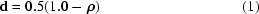or a distance-squared matrix, D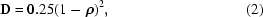for each entry i,j in d, 0.0 ≤ dij ≤ 1.0. A correlation coefficient of 1.0 translates to a distance of 0.0, a coefficient of −1.0 to 1.0, and zero to 0.5. There are other methods of generating a distance matrix from ρ (see, for example, Gordon, 1981), but we have found this to be as effective as any other.

For some purposes we also need a dissimilarity matrix S, the elements of which are defined via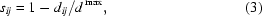where d max is the maximum distance in matrix d.

#### 2.2. Cluster analysis

Using d, we can now carry out agglomerative hierarchical cluster analysis to put the patterns into classes as defined by their distances from each other. [Gordon (1981, 1999) provides an excellent and detailed introduction to the subject; note that the two editions of this monograph are quite different, yet complementary; the first edition is especially recommended as an introductory text.] We begin with a situation in which each pattern is considered to be in a separate class. We then search for the two patterns with the shortest distance between them, and join them into a single cluster. This continues in a stepwise fashion until all the patterns form a single cluster. When two classes (Ci and Cj) are merged, there is the problem of defining the distance between the newly formed class CiCj and any other class Ck. There are a number of different ways of doing this, and each one gives rise to a different clustering of the patterns, although often the difference can be quite small. A general algorithm has been proposed by Lance & Williams (1967) and is summarized in a simplified form by Gordon (1981), as is shown in Table 1. The distance between the new class formed by merging Ci and Cj, and any other class Ck is given by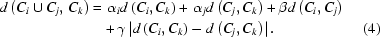There are a considerable number of possible clustering methods. Table 1defines six clustering methods that we have found useful, defined in terms of the parameters α, β and γ. All these methods can be used with powder data, although, in general, we have found the group average link or single-link formalism to be the most effective.

 Table 1A general algorithm as proposed by Lance & Williams (1967)and summarized in a simplified form by Gordon (1981)The distance between the new class formed by merging clusters Ci and Cj, and any other class Ck is given by d(Ci ∪ Cj, Ck) = αid(Ci, Ck) + αid(Cj, Ck) + βd(Ci, Cj) + γ|d(Ci, Ck) − d(Cj, Ck)| and the table classifies the methods according to the coefficients αi, β and γ. nj is the number of members in cluster j, etc.
Method αi β γ
Average link ni/(ni + nj) 0 0
Weighted average link ½ 0 0
Centroid ni/(ni + nj) ninj/(ni + nj)2 0
Sum of squares (ni + nk)/(ni + nj + nk) nk/(ni + nj + nk) 0

The results of cluster analysis are usually displayed as a dendrogram, a typical example of which is shown in Fig. 1(a) where a set of 21 powder patterns is analysed using the complete-link method. Each pattern begins at the bottom of the plot as a separate class, and these amalgamate in stepwise fashion, linked by horizontal tie bars. The height of the tie bar represents a similarity measure as measured by the relevant distance. As an indication of the differences that can be expected in the various algorithms used for dendrogram generation, Fig. 1(b) shows the same data analysed using the single-link method: the resulting clusterings are slightly different, there is one less cluster and the similarity measures are larger, and, as a consequence, the tie bars are lower on the graph.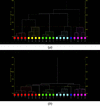Figure 1 A typical dendrogram for a set of 21 powder diffraction patterns (a) using the complete-link method and (b) the single-link method on the same data.

#### 2.3. Principal-component analysis

We can also carry out principal-component analysis (PCA) on the correlation matrix. The eigenvalues of the correlation matrix can be used to estimate the number of clusters present via a scree plot (see §2.5), and the eigenvectors can be used to generate a score plot which can be used as a visualization tool to indicate which patterns belong to which class. Score plots traditionally use two components with the data thus projected onto a plane (see, for example, MINITAB, 2003); we use three-dimensional plots in which three components are represented. Visualization in this way is discussed further in §3.

#### 2.4. Metric multidimensional scaling

Given an n × n distance matrix dobs, metric multidimensional scaling (MMS) seeks to define a set of p underlying dimensions that yield a Euclidean distance matrix, dcalc, the elements of which are equivalent to, or closely approximate the elements of dobs. It is very much like solving a Patterson map, where we have a set of vectors generating a distance matrix, and we are trying to extract a set of underlying atomic coordinates before the application of rotation and translation functions (in this case p = 3).

The method works as follows (Gower, 1966).

The matrix dobs has zero diagonal elements, and so is not positive semidefinite. A positive definite matrix, A(n × n), can be constructed, however, by computing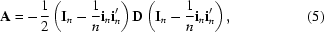where In is an (n × n) identity matrix, in is an (n × 1) vector of unities, and D is defined in equation (2). The matrix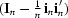is called a centering matrix since A has been derived from D by centering the rows and columns.

The eigenvectors v1, v2, … vn and the corresponding eigenvalues λ1, λ2, … λn are then obtained. A total of p eigenvalues of A are positive and the remaining (np) will be zero. For the p non-zero eigenvalues, a set of coordinates can be defined via the matrix X(n × p)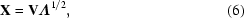where Λ is the vector of eigenvalues.

If we now set p = 3, then we are working in three dimensions and the X matrix can be used to plot each pattern as a single point in a three-dimensional graph. This assumes that we can reduce the dimensionality of the problem in this way and still retain the essential features of the data. As a check, we can compute a distance matrix dcalc from X(n × 3) and compare it with the observed matrix dobs using both the Pearson and Spearman correlation coefficients. In general, the MMS works well and correlation coefficients >0.95 are common. For large data sets this can reduce to ca 0.6, which is still sufficiently high to suggest the viability of the procedure. There are occasions when the underlying dimensionality of the data is 1 or 2, and in these circumstances the data project onto a plane or a line in an obvious way without any problems.

#### 2.5. Estimating the number of clusters

Estimating the number of clusters is an unsolved problem in classification methods. We use two approaches: (a) eigenvalue analysis of matrices ρ and A, and (b) those based on cluster analysis.

Eigenvalue analysis is well understood: the eigenvalues of the relevant matrix are sorted in descending order and when a fixed percentage (we typically use 95%) of the data variability has been accounted for, the number of eigenvalues is selected.

We carry out eigenvalue analysis on the following.

(i) Matrix ρ as described in §2.3.(ii) Matrix A as described in §2.4.(iii) A transformed form of ρ in which ρ is standardized to give ρs in which the rows and columns have zero mean and unit variance. The matrix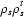is then computed and subjected to eigenanalysis. This procedure is used, for example, in the MINITAB statistics software (MINITAB, 2003). It tends to give a lower estimate of cluster numbers.

Methods based on clustering are less well known in crystallography. What is sought here is a stopping rule where we seek to define the number of clusters in the data set. In terms of the dendrogram, this is equivalent to `cutting the dendrogram', i.e. the placement of a horizontal line across the dendrogram such that all the clusters as defined by tie lines above this line remain independent and unlinked. The most detailed study is that of Milligan & Cooper (1985), summarized by Gordon (1999), and from this we have selected three tests as follows, which seem to operate effectively with powder data.

(iv) The Calinski & Harabasz (1974) (CH) test: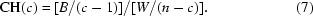A centroid is defined for each cluster. W denotes the total within-cluster sum of squared distances about the cluster centroids, and B is the total between-cluster sum of squared distances. Parameter c is the number of clusters chosen to maximize equation (7).

(v) A variant of Goodman & Kruskal's γ test (1954) as described by Gordon (1999). The dissimilarity matrix as defined in equation (3)is used. A comparison is made between all the within-cluster dissimilarities and all the between-cluster dissimilarities. Such a comparison is marked as concordant if the within-cluster dissimilarity is less than the between-cluster dissimilarity, and discrepant otherwise. Equalities, which are unusual, are disregarded. If S+ is the number of concordant comparisons and S the number of discrepant comparisons, then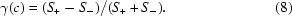A maximum in γ is sought by an appropriate choice of cluster numbers.

(vi) The C test (Milligan & Cooper, 1985). We choose the value of c that minimizes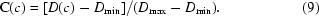D(c) is the sum of all the within-cluster dissimilarities. If the partition has a total of r such dissimilarities, then Dmin is the sum of the r smallest dissimilarities and Dmax the sum of the r largest.

Tests (iv), (v) and (vi) depend on the clustering method that is being used. To reduce the bias towards a given classification scheme, these tests are carried out on four different clustering methods: the single-link, the group-average, the sum of squares and the complete-link methods. Thus we have 12 semi-independent estimates of the number of clusters from clustering methods, and three from eigenanalysis, making 15 in all.

We use a composite algorithm to combine these estimates. The maximum and minimum values of the number of clusters (cmax and cmin, respectively) given by the eigenanalysis results [(i)–(iii) above] define the primary search range; tests (iv)–(vi) are then used in the range max(cmin − 3, 0) ≤ c ≤ min(cmax + 3, n) to find local maxima or minima as appropriate. The results are averaged, any outliers are removed, and a weighted mean value of the remaining indicators is taken and used as the final estimate of the number of clusters.

A typical set of results is shown in Fig. 2and Table 2. The scree plot arising from the eigenanalysis of the correlation matrix indicates that 95% of the variability can be accounted for by five components, and eigenvalues from other matrices indicate that four clusters are appropriate. A search for local optima in the CH, γ and C tests is then initiated in the range of 2–8 possible clusters. Four different clustering methods are tried, and the results indicate a range of 4–7 clusters. There are no outliers, and the final weighted mean value of five is calculated. As Fig. 2(a) shows, the optimum points for the C and γ tests are often quite weakly defined. Confidence levels for c are defined by the estimates of the maximum and minimum cluster numbers after any outliers have been removed.

 Table 2Estimating the number of clusters present for the pharmaceutical data used for Figs. 2and 3NA implies that no optimum point could be found in the required range. The maximum estimate of the number of clusters is 7; the minimum estimate is 4; the median value is 5, and the combined weighted estimate of the number of clusters is 6 with confidence limits 4–7.
Method No. of clusters
Principal components analysis (non-transformed matrix) 5
Principal components analysis (transformed matrix) 4
Multidimensional metric scaling 4
Gamma statistic using single linkage 7
Calinski–Harabasz statistic using single linkage 7
C statistic using single linkage NA
Gamma statistic using group averages 7
Calinski–Harabasz statistic using group averages 5
C statistic using group averages NA
Gamma statistic using sum of squares NA
Calinski–Harabasz statistic using sum of squares 5
C statistic using sum of squares NA
Gamma statistic using complete linkage NA
Calinski–Harabasz statistic using complete linkage 5
C statistic using complete linkage NA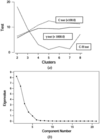Figure 2 Four different methods of estimating the number of clusters present in the data: (a) the use of the C test [the C(c) coefficients have been multiplied by 100.0], the γ test (coefficients × 10.0), and the CH test; (b) a scree plot from the eigenanalysis of the correlation matrix.

#### 2.6. Choice of clustering method

It is possible to use the metric multidimensional scaling (or, alternatively, PCA score plots) to assist in the choice of clustering method, since the two methods operate independently. The philosophy here is to choose a technique which results in the tightest, most isolated clusters, as follows.

(i) MMS is used to derive a set of three-dimensional coordinates stored in matrix X(n × 3)

(ii) The number of clusters, c, is estimated as in the previous section.

(iii) Each of the six dendrogam methods is employed in turn, stopping when c clusters have been generated. Each entry in X can now be assigned to a cluster.

(iv) Draw a sphere around each point in X and calculate the average between-cluster overlap of the spheres for each of the N clusters C1 to CN. If the total number of overlaps is m, we can write this as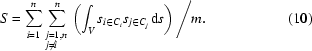If the clusters are well defined then S should be a minimum. Conversely, poorly defined clusters will tend to have large values of S. In the algorithm we use, the sphere size depends on the number of diffraction patterns.

(v) The individuality of each cluster is also estimated by computing the mean within-cluster distance. This should also be a minimum for well defined, tight clusters.

(vi) We also compute the mean within-cluster distance from the centroid of the cluster.

(vii) Steps (iv)–(vi) are repeated using coordinates derived from PCA three-dimensional score plots.

(viii) Tests (iv)–(vii) are combined in a weighted, suitably scaled mean to give an overall figure of merit (FOM); the minimum is used to select the dendrogram method to be employed.

The same formalism can be used to decide which of the MMS- or PCA-based three-dimensional plots is likely to represent the data best. The final FOM is computed for both the PCA and MMS methods; the lowest is used as the indicator.

Table 3shows the methodology at work. Table 3(a) uses equation (10)on the MMS- and PCA-derived matrices X. At this stage, the single-link method is preferred for clustering, and the PCA formalism for presenting the data in three dimensions. Table 3(b) is based on mean intra-cluster distances and again the single-link method is the choice for clustering, but the MMS method is preferred for data presentation. Table 3(c) repeats the calculations of 3(b) with the same outcome. All these results are combined in Table 3(d). As a result the PolySNAP program selects the single-link method as the optimum clustering method for generating dendrograms for these data. In addition, MMS is predicted to give the best three-dimensional plots.

 Table 3Establishing the best clustering method: (a) calculations using MMS and PCA based on cluster overlap; (b) calculations based on mean intra-cluster distances; (c) calculations based on centroid–cluster distances; (d) combination of (a)–(c)
 (a)
Clustering strategy MMS PCA
Centroid method 8.144 6.834
 (b)
Clustering strategy MMS PCA
Centroid 3.998 3.639
 (c)
Clustering strategy MMS PCA
Centroid 3.123 4.510
 (d)
Clustering strategy Figure of merit MMS PCA
Weighted average link 4.292 3.902 4.682
Centroid 5.041 5.088 4.994
Group average link 4.292 3.902 4.682

#### 2.7. The most representative sample

Similar techniques can be used to identify the most representative sample in a cluster. We take this to be that sample which has the minimum mean distance from every other sample in the clusters, i.e. for cluster J containing m patterns, the most representative sample, i, is defined as that which gives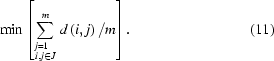The most representative sample is useful in visualization (§3) and generating a database of known phases (§5.2).

#### 2.8. Mixtures

In paper (I) we have shown how mixtures may be subjected to quantitative analysis using a least-squares algorithm based on the use of singular value decomposition in the matrix inversion procedures. The same formalism is valid here. If quantitative analysis is required, a database of known pure phases is created and input into the procedure. Every sample is checked against the reference database. If significant correlations are not found, a mixture is suspected and a quantitative analysis is carried out as in §5 of paper I. The quality of data that result from high-throughput crystallography makes it unlikely that an accuracy better than 5–10% can be achieved, but nonetheless, the identification of mixtures is an important and necessary part of high-throughput experiments, and this procedure can provide useful indications, as shown in §§5.2and 5.4.

#### 2.9. Amorphous samples

Amorphous samples are an inevitable consequence of high-throughput experiments and need to be handled correctly if they are not to induce erroneous clustering indications. In our procedures, we estimate the total background for each pattern and integrate its intensity; we also calculate the integrated intensity of the non-background signal. This is independent of background removal. If the ratio falls below a pre-set limit (usually 5%, but this may vary with the type of sample under study) the sample is treated as amorphous. The distance matrix is then modified so that each amorphous sample is given a distance and dissimilarity of 1.0 from every other sample, and a correlation coefficient of zero. This automatically excludes the samples from the clustering until the last amalgamation steps, and also limits their effect on the eigenanalysis and hence the estimation of the number of clusters.

### 3. Data visualization

It is important when dealing with large data sets to have suitable visualization tools. This methodology provides four such aids.

(a) The dendrogram gives the clusters, the degree of association within the clusters and the differential between a given cluster and its neighbours. Different colours are used to distinguish each cluster. The cut line is also drawn, along with the confidence levels.

(b) The MMS method reproduces the data as a three-dimensional plot in which each point represents a single powder pattern. The colour for each point is taken from the dendrogram. The most representative sample for each cluster is marked with a cross.

(c) Similarly, the eigenvalues from principal-component analysis can be used to generate a three-dimensional score plot in which each point also represents a powder pattern. Just as in the MMS formalism, the colour for each point is taken from the dendrogram and the most representative sample is marked.

(d) Finally, a well chart is produced for each sample, corresponding to the sample wells if relevant, in which each well is given a colour as defined by the dendrogram. If mixtures of known phases are detected, the pie charts give the relative proportions of the pure samples as estimated by quantitative analysis.

Features (a)–(d) give an easy to manipulate graphical view of the data, which are semi-independent, and thus can be used to check consistency and discrepancies.

### 4. The procedure

We can now define the full analysis procedure.

(i) The data are imported. As described in paper (I), each pattern is interpolated or extrapolated to give 0.02° increments in 2θ. Data are normalized, backgrounds are optionally removed; wavelets are optionally used to smooth the data, and the peaks identified. (It is worth remembering that this latter step, in general, is not required unless peak-specific statistics are to be employed.)

(ii) A correlation matrix is generated in which the full profile of every pattern in a set of n patterns is matched with every other to give an n × n correlation matrix ρ using a weighted mean of the Spearman and Pearson correlation coefficients with the optional inclusion of the Kolmogorov–Smirnov and Pearson peak correlation tests. The latter two tests require peak positions. An optimal shift in 2θ between patterns is often required, arising from equipment settings, especially the sample height, and data collection protocols. In paper (I), we use the form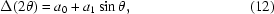where a0 and a1 are constants adjusted to maximize pattern correlation.

(iii) The correlation matrix is examined for stability in eigenanalysis and cluster analysis using singular value decomposition.

(iv) Amorphous samples are identified and isolated from the calculations, although not wholly excluded.

(v) If a database of pure phases is present, quantitative analysis may be carried out on each sample if the correlation is not sufficiently large.

(vi) Eigenanalysis is carried out to give the principal indicators of the number of clusters. This is followed by a search for local optima in the CH, γ and C tests. Outliers are removed and a weighted mean estimate with confidence limits is defined.

(vii) The optimal clustering method is established as outlined in §2.6and a dendrogram generated.

(viii) The most representative sample of each cluster is identified.

(ix) Visualization as described in section §3is carried out.

All these steps are performed in a program called PolySNAP (Barr et al., 2003) which runs on a PC under Windows 2000 or XP. Contained within this software is the SNAP-1D program (Barr et al., 2003). Although the calculation is elaborate, the total time taken on a 2.4 GHz PC varies between <1 min for 100 samples and ca 1 h for 1000. The rate-determining step in the computations is the use of clustering methods to determine the number of clusters: some of the methods used are of order n3 in time and so become very significant with large samples. Computing times are considerably increased if optimal shifts [equation (12)] are estimated.

It is important to note that no one method is optimal in these calculations, and that a combination of mathematical and visualization techniques is required, which often needs tuning for each individual application. §5.3presents an example of this.

### 5. Examples

Three test data sets are used in this paper to demonstrate differing aspects of the methodology.

(a) A proprietary pharmaceutical compound using data on five chosen polymorphs collected on a Bruker D8-GADDS system.

(b) Commercial aspirin tablets for which thirteen samples of aspirin tablets as supplied by pharmacies were used; data were collected on a Bruker D8 diffractometer.

(c) A database of 19 patterns comprising a subset of the ICDD database set 78 (ICDD, 2003). The peaks as listed were used to generate a set of profile data assuming pseudo-Voigt peak profile shapes. Synthetic mixtures of various components of this database were used. Although these data are, in part, artificial, they are useful in exploring the limits of cluster analysis and mixture detection.

All the samples are relatively small, so that they can easily be presented graphically and discussed. Examples of larger data sets with over 1000 patterns will be published elsewhere.

#### 5.1. Polymorphs

A data set comprising 21 pharmaceutical samples, as described above, was collected on a Bruker D8-GADDS system and examined. Five polymorphs were expected. The dendrogram is shown in Fig. 1(a). The group single-link method was used for generating this. The associated pie chart is in Fig. 3(a), the MMS plot in Fig. 3(b), and the three-dimensional PCA score plot in Fig. 3(c). It was estimated that there were six clusters present.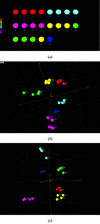Figure 3 (a) The pie chart corresponding the dendrogram in Fig. 1(a); (b) the corresponding three-dimensional MMS plot; (c) the PCA scores plot in three dimensions.

In general, the data are consistent: the dendrogram forms six distinct well differentiated clusters, and this is matched by the MMS and three-dimensional score plots where the clusters are also clearly defined. The well chart gives a useful summary of well contents. Patterns 20 and 21 form singleton clusters in the dendrogram. In the three-dimensional plot, pattern 21 is quite isolated; pattern 20 is, however, quite close to another cluster of seven patterns so that it is not quite clear whether it is a single sample, or if it involves mixtures involving components from the other five clusters. Similarly, it would be useful to know if pattern 21 is a pure phase. The application of quantitative analysis can assist here.

#### 5.2. Quantitative analysis of the polymorph data

The above data were reprocessed but, in this case, a reference database was generated by using the most representative sample of each of the five clusters that contained more than one member. The results from PolySNAP are the same as in §5.1except the pie charts for samples 20 and 21 now identify them as mixtures (Fig. 4). The remaining samples are still identified as pure phases. The five expected polymorphs for this data set have now been clearly identified using less than 1 min of computing time.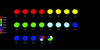Figure 4 The pie chart corresponding to Fig. 3but using a database of the most representative samples as a reference. Patterns 20 and 21 can be seen to correspond to mixtures.

#### 5.3. Aspirin data

This example shows the method and the program used in a slightly more sophisticated and less automatic way. The 13 powder data sets, after processing by PolySNAP, are shown in Fig. 5arranged into groups based on similarity. Because we are dealing with such a small data set, this is easily done; it becomes impossible with larger data sets. The samples were input into PolySNAP in automatic mode. The resulting dendrogram, pie chart, MMS and score plots are shown in Fig. 6. Four clusters have been identified in the dendrogram and these have been appropriately coloured. However, inspection of the three-dimensional plots, where the dendrogram colours are used, indicates that the samples represented in red would appear to form two distinct classes, thus giving rise to five groups in total instead of four. A new cut point for the dendrogram was selected to reflect this. The revised graphical output is shown in Fig. 7. It can be seen that this partitioning of the data now fully reflects the raw diffraction data.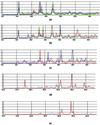Figure 5 The powder patterns for the 13 commercial aspirin samples partitioned into five sets. The patterns are in highly correlated sets: (a) comprises patterns 1, 3, 5, 6, 9, 12, (b) comprises 10, 11, 13, (c) contains patterns 2 and 4, (d) contains sample 7, and (e) sample 8.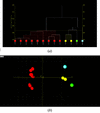Figure 6 (a) The initial default dendrogram for 13 aspirin samples. The data are partitioned into four clusters. (b) The corresponding MMS plot. The red cluster has a natural break or partition in it.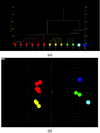Figure 7 (a) The dendrogram in Fig. 6is now cut so that there are five clusters corresponding to the groups in Fig. 5. (b) The corresponding MMS plot. The red cluster in Fig. 6(b) is now partitioned into two distinct clusters.

This mode of use of PolySNAP is common. The difficulties of unambiguously determining the number of clusters means that user inspection using appropriate visualization tools can often be helpful.

As a demonstration of the handling of amorphous data, five amorphous patterns as shown in Fig. 8(a) were included in the aspirin data and the clustering calculation repeated. The results are shown in Fig. 8(b). Fig. 8(c) shows the corresponding pie chart. It can be seen that the amorphous samples are positioned as isolated clusters on the right-hand end of the dendrogram. It could be argued that these samples should be treated as a single five-membered cluster rather than five individuals, but we have found that this confuses the clustering algorithms and it is clearer to the user if the amorphous data are presented as separate classes.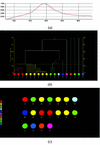Figure 8 The aspirin data into which five amorphous data samples have been incorporated. (a) A typical amorphous pattern as incorporated into the data. (b) The resulting dendrogram with the amorphous samples isolated at the right-hand end. (c) The corresponding pie chart; the amorphous samples are clearly indicated.

#### 5.4. Inorganic mixtures

A database of 19 patterns from set 78 of the ICDD database for inorganic compounds (ICDD, 2003) was imported into the program. To this was added some simulated mixture data generated by adding the patterns for lanthanum strontium copper oxide and caesium thiocyanate diffraction data in the proportions 80/20, 60/40, 50/50, 40/60 and 20/80%, respectively. Two calculations were performed: an analysis without the pure-phase database and a second where the pure phases of lanthanum strontium copper oxide and caesium thiocyanate were present.

The results are shown in Fig. 9. In the MMS plot the green spheres represent pure lanthanum strontium copper oxide, while the yellow are pure caesium thiocyanate. The red spheres represent mixtures of the two. The latter form an arc between the green and yellow clusters. The distance of the spheres representing mixtures from the lanthanum strontium copper oxide and caesium thiocyanate spheres gives a semi-quantitative representation of the mixture contents. Running the program in quantitative mode gives the pie charts also shown in Fig. 8; they reproduce exactly the relative proportions of the two components.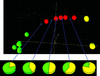Figure 9 Identifying mixtures using lanthanum strontium copper oxide and caesium thiocyanate diffraction data taken from the ICDD database, set 78, using pseudo-Voigt peak profiles. The green spheres represent pure phases of lanthanum strontium copper oxide and the yellow pure caesium thiocyanate. The red spheres represent mixtures of the two in the relative proportions of lanthanum strontium copper oxide/caesium thiocyanate 80/20, 60/40, 50/50, 40/60 and 20/80%, respectively in an arc commencing on the left-hand side of the diagram. The pie charts give the results of an independent quantitative calculation in which lanthanum strontium copper oxide and caesium thiocyanate have been included as pure phases in a reference database.

### 6. Conclusions

We have shown that the use of parametric and non-parametric matching techniques can generate a correlation matrix which can be converted to distance and dissimilarity forms, which can then be input into cluster analysis, multivariate data analysis and related visualization techniques to identify the natural groupings of the patterns. The method is viable for at least 1000 data sets. It can also provide an approximate estimate of the components of quantitative mixtures when reference patterns are present. These techniques are especially valuable in high-throughput situations (although, as we shall show in other papers, they can be very useful with small data sets as well). It is important to have available as wide a range of techniques as possible for exploring such data, because no single method is adequate for the task and the methods need to be used together. The methods are incorporated in the commercial software PolySNAP, licensed to Bruker-AXS.

Clustering and multivariate analysis are large subjects with an extensive literature, and this paper has only touched upon a few methods relevant to the problem of classifying powder patterns. We are currently investigating other areas of data analysis, including fuzzy clustering (Sato et al., 1997) and silhouettes (Rousseeuw, 1987), which can both be used as semi-independent methods for identifying samples which may be mixtures of other clusters. We are also using minimum spanning trees (see, for example, Graham & Hell, 1985) as an interactive way of exploring the links between clusters and their members. The results will be published at a later date.

Of course, this method should work with any one-dimensional data set, although data with very sharp peaks pose problems because correlations can rapidly fall to very small values unless there is exact peak overlap. Techniques which should be amenable to this approach include Raman, IR and solid-state NMR spectroscopies, and DSC. Preliminary tests on Raman and IR data have proved encouraging.

### Acknowledgements

We wish to thank Bob Docherty, Chris Dallman, Richard Storey, Neil Feeder and Paul Higginson of Pharmaceutical Sciences, Pfizer Global R and D, UK, for data, many useful discussions and suggestions, and for pioneering and supporting this project; the ICDD (especially John Faber) for access to the ICDD database, and Arnt Kern and Stefan Haaga at Bruker-AXS for the aspirin data; and finally Laura Hamill for the calculations on the lanthanum strontium copper oxide, caesium thiocyanate mixtures.

### ReferencesJOURNAL OFAPPLIEDCRYSTALLOGRAPHY